# Schrödinger representation

One of the basic possible (together with the Heisenberg representation and the interaction representation (cf. Interaction, representation of)) equivalent representations of the dependence on timeof operatorsand wave functionsin quantum mechanics and quantum field theory. In the Schrödinger representation the operatorscorresponding to physical dynamical quantities do not depend on; thus, the solution of the Schrödinger equation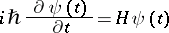(1)

can be formally expressed by the Hamilton operator, which is independent of, in the form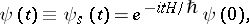(2)

where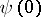, being the initial value, does not depend on time, and the wave function in the Schrödinger representation depends onand contains all information with respect to changes in the state of the system whenchanges. The mean value of the operator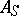in the Schrödinger representation(3)depends onas a result of the dependence onof the wave functions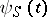.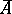can be also considered as the mean value of the time-dependent operator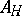over the wave functions, which do not depend on: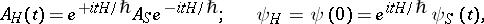(4)

i.e. as the mean value of an operator in the Heisenberg representation. The invariance property of the mean value (which should be observable and have physical meaning) under unitary transformations of type (4) means that the Schrödinger representation, the Heisenberg representation and the interaction representation are equivalent.

The Schrödinger representation was called after E. Schrödinger, who introduced it in 1926 when formulating an equation in quantum mechanics that was later called the Schrödinger equation.